Doppler effects and their physical causes

The following is a brief explanation of the Doppler effect and its impact on the plausibility of the law of constant light speed, c. We will define the Doppler effect as "the change in pitch or frequency of energy-containing oscillations received from a source."

Causes of Doppler effect in a medium The causes of Doppler shifts in a medium (e.g. air, water, aluminum) are well understood.  Doppler effects involve the rate at which energy waves in a medium arrive at an observer (e.g. human ear, microphone).  The rate is generally referred to as the "pitch" or "frequency," and we will represent this quantity by the symbol, f.  The pitch or frequency can be specified in "cycles per second" or "hertz," which is designated by the symbol, Hz.  A sound of 1000 Hz (1 kHz) at the observer means that 1000 times a second an oscillation of the medium at the observer occurs due to multiple causes.  One cause is the oscillation frequency of the source of the energy (e.g. human vocal system, electromechanical speaker) imparted to the medium.  Sound energy waves are propagated through the air from a source to an observer, and the speed of propagation through the air depends on the properties of the air (e.g. temperature, humidity).

The Doppler effect in air will be discussed first.  The explanation will involve a source of sound, S, a medium, M, through which the sound propagates, and an observer, O, who hears the sound.  The figure below shows the source and observer located along the x axis of a coordinate system at rest relative to the medium, M.  It is important to realize that it is waves of energy in the form of air pressure differences in M that are moving from S to O.  Molecules in the air are not moving from S to O.The medium, M, has properties that result in a constant speed of sound propagation, vp, through M of 340 m/s (340 meters per second).  Therefore, vp=340 m/s, as specified in the figure.  We will also assume that source, S, emits energy into the medium with a constant emission frequency of 340 Hz.  We will designate this constant oscillation frequency of the source, f s.  Therefore, f s=340 Hz, as shown.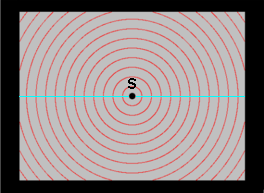When S is at rest in M If S is "at rest" in M (i.e. not moving through M) then the speed of the sound waves caused by S move away from S in every direction with a speed relative to S of 340 m/s.  Along a line from S in any direction, the distance between successive locations of maximum pressure (or minimum pressure) in the sound waves moving through M is 1 m.  In the figure, the radial distance from one red line to the next is 1 m.  This distance is the "wavelength," and this wavelength will be designated by the symbol, λ (lambda). Therefore, λ=1 m when S is at rest in M, and λ is isotropic in M.  An observer at rest anywhere in M will hear the real, actual frequency emitted by S because no Doppler shift occurs at either the source or the observer.  The frequency of the sound heard by the observer, fo, is the same as the 340 Hz frequency of the oscillations of the source, f s.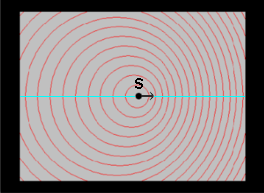When S is moving through M In this figure, the sound waves caused by a speaker, or other means of creating energy waves in the air, are again moving at the same speed away from the locations where the waves are created.  But due to the motion of S, each successive wave is displaced in the +x direction from the previous wave, as shown.  This causes the waves moving in the +x direction to be closer together and therefore shorter.  The shorter waves arrive at an observer at rest on the x axis in the +x direction from S with a frequency higher than f s.  Conversely, an observer at rest in the −x direction from S would receive energy waves with a frequency lower than f s.  In both of these cases, when S is moving through the medium, a Doppler shift occurs, and it is caused at the source, not at the observer.  In both cases the sound waves arrive at the observer with the same 340 m/s speed relative to the observer.
When S is moving along the x axis, the Doppler shift at S depends on the velocity of S through the medium (i.e. relative to the medium).  We will designate this velocity vSM, where vSM toward an observer, O is positive and vSM away from O is negative. We can see that as vSM approaches 340 m/s, all the waves moving through M from S to O will be very close together in front of S and will reach observer O, who is on the x axis ahead of S, at nearly the same time, making λ very short, the frequency very high, and the sound at O a loud, high-pitch clap as S passes O.  Therefore, for any value of vSM, we might expect that the frequency of the sound arriving at any point on the x axis in reference frame M, fM, will be specified by the following equation (which appears correct because, when vSM=170 m/s, the equation yields 680 Hz for an observer ahead of S and 226.666 Hz for an observer behind S).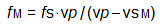When observer, O, is moving through M toward or away from S
The velocity of observer, O, along the x axis in M will be designated vOM, where vOM away from the source, S, is positive and vOM toward S is negative.  When vOM is negative (i.e. when the direction of vOM through M is opposite to the direction of the approaching sound waves propagating through M, vp), the velocity of the sound waves relative to O, designated vWO, is greater than vp, and the frequency at which the waves arrive at O, fo, is increased due to vOM.  And when vOM is positive (i.e. when the direction of vOM is the same as the direction of vp), vWO and fo are less than vp and fM respectively due to vOM (if vOM < vp).  The ratio of the observed frequency as a result of vOM≠0 to the observed frequency when vOM=0 is specified as follows.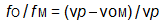Therefore, when vp and vOM have the same magnitude and direction, vOM is positive, (fo /fM) = 0, and observer, O, does not hear any sound from S.  And when vp and vOM have the same magnitude but opposite direction, vOM is negative and (fo /fM) = 2, in which case the speed of the sound waves relative to the observer (vWO) is twice the speed of sound through the medium, and fo is twice what it would be if O were at rest on the x axis.

Combining the Doppler shift equations
We can combine the above equation for determining the frequency, fM, heard by an observer at rest at any point on the x axis, and the equation for the frequency ratio due to O's motion toward or away from S.  The following combination specifies the frequency heard by an observer on the x axis when the observer's velocity is vOM and the source's velocity is vSM.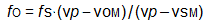Table of Doppler shift causes
The following table summarizes the various causes of Doppler shifts discussed above.  It helps understand that Doppler shifts can occur at the source and at the observer due to the motions of S and /or O through M.  The table reflects what the above equation specifies, namely the causes responsible for the change in frequency from f s to fo.  For example, the table cell in the "Observer, O, at rest in M" column and in the "Source, S, moving in M" row shows a change in frequency from source, S, to medium, M, due to the velocity of S relative to M, vSM, being unequal to zero and the resulting velocities of the energy waves leaving S, vWS, being unequal to the velocity of propagation, vp of the energy waves through M.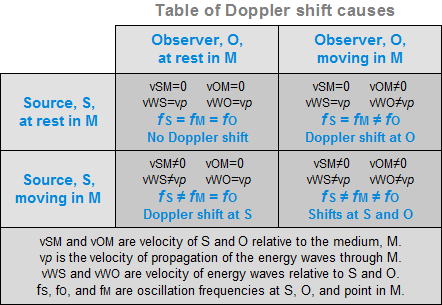Doppler shifts of electromagnetic energy oscillations in the quantum medium (qm)
Several modifications of the above equation will make it able to determine Doppler shifts of light frequencies.  Dividing both sides by f s makes them the ratio of fo to f s.  And expressing all velocities in terms of the constant, absolute speed of light, ca, through the qm makes vp=1 ca, as shown in the following equation.  Also, to be consistent with the qm view's symbols, absolute velocities through the qm, vSM and vOM will be written as vSa and vOa respectively.  The physical change ratios, rvSa and rvOa, must also be included to account for the slowing of all processes at S and O due to their velocities through the qm.  (The slower processes of interest are the lower frequencies of the photons emitted at S and the slower clocks at O, which make the observed, virtual frequency of the light arriving from S higher than if the clocks at O were keeping time in absolute seconds.)  These physical change ratios for S and O are rvSa=sqrt(1− vSa) and rvOa=sqrt(1− vOa).  The following equation specifies exactly the same fo /f s ratio as specified by the customary, orthodox, relativistic Doppler shift equation to be discussed below.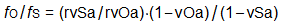This equation can be simplified by making it for the observer's reference frame and for the virtual phenomena observed in this frame.  In this frame (where observers assume that the light speed from S to O is c and that photons are not propagated through a medium) the variable vOa is eliminated, as are (1+ vOa) and rvOa.  The velocity of the source relative to the qm, vSa becomes vSO, the observed, virtual velocity of S relative to O.  And the physical change ratio for the source, rvSa, is replaced by the virtual physical change ratio between the source and observer, rvSO.  The virtual relative velocity, vSO, and virtual physical change ratio, rvSO, are specified by the following equations (as explained in more detail on the Equations page).  vSO=(vSa−vOa) / (1− vSa·vOa) ,  rvSO=sqrt(1− vSO).  The end result is the following simple equation that specifies exactly the same fo /f s ratio as specified by the above equation and by the customary relativistic Doppler shift equation.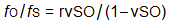Why orthodox physics theory cannot explain the Doppler shifts of light The qm view explains the physical causes for the Doppler redshifts and blueshifts of light the same way Doppler shifts of sound energy are explained above.  This is possible because, in the qm view, light is comprised of oscillations in a medium that propagate through the medium at a speed that depends on the properties of the medium (much as sound is oscillations in a medium that propagate through a medium at speeds that depend on the medium).  Doppler shifts of light occur at the source due to the source's motion through the qm (i.e. its absolute velocity), and they occur again at the observer due to the observer's absolute velocity.  If light is not propagated through a medium, how can the Doppler shifts of light occur?

Also, how can the Doppler shifts of light occur if, as orthodox physics assumes, the speed of light leaving a source S is always the same speed, c, and the speed of light arriving at the observer O is also c, and never changes when O's velocity changes?  Orthodox theory cannot answer this question, and it appears that people have learned to ignore this fundamental problem with orthodox theory.  The following paragraph is an example from a usually-reliable information source.
http://en.wikipedia.org/wiki/Relativistic_Doppler_effect (as of 02/01/14)
 Understanding relativistic Doppler effect requires understanding the Doppler effect, time dilation, and the aberration of light.  As a simple analogy of the Doppler effect, consider two people playing catch.  Imagine that a stationary pitcher tosses one ball each second (1 Hz) at one meter per second to a catcher who is standing still.  The stationary catcher will receive one ball per second (1 Hz).  Then the catcher walks away from the pitcher at 0.5 meters per second and catches a ball every 2 seconds (0.5 Hz).  Finally, the catcher walks towards the pitcher at 0.5 meters per second and catches three balls every two seconds (1.5 Hz).  The same would be true if the pitcher moved toward or away from the catcher.  By analogy, the relativistic Doppler effect shifts the frequency of light as the emitter or observer moves toward or away from the other.
This analogy ignores the fact that the speed of the balls relative to the catcher is not constant.  The speed changes whenever the pitcher or catcher change their motion toward or away from the other.  The speed of the balls relative to the catcher ranges from .5 m/s to 1.5 m/s.  Therefore, the analogy is not like a source and observer of light where, according to orthodox physics theory, the photons arrive with the same speed, c, relative to the observer regardless of the motions of the source or the observer toward or away from the other.  What can explain the fact that people can ignore this incompatibility between constant light speed, c, and Doppler shifts of light, and not recognize that the incompatibility is a serious problem for any theory that is based on light speed, c?

The qm view explains why the speed of light leaving a source and arriving at an observer is always the same speed, c, but the explanation is complex.  Briefly, c is the virtual speed of light that is measured and observed in every inertial reference frame.  It is caused by physically distorted time- and distance-measuring instruments in all inertial frames due to their velocities through the qm.  Remarkably, the distorted time and distance measurements in an inertial frame always result in measured light speed, c.  An understanding of the qm view allows distorted, virtual times and distances to be converted into absolute times and distances that reveal the real, absolute speeds of light relative to a source S and relative to an observer O that cause the observed Doppler shifts.

Is it not very apparent that Doppler shift phenomena fits perfectly with the qm view and is also impossible if light is not propagated through a medium or if photons always have the same speed relative to all sources and observers?

Orthodox physics theory assumes that light is not propagated through a medium because the Michelson-Morley experiment and similar experiments showed this assumption to be correct.  Experiments also showed that light moves with a speed that is somehow constant relative to all inertial observers in remote space.  The qm view explains (via printed documents, webpages, and videos) the physical causes for these misleading experimental results.  A simple example showing the causes of constant light speed, c, in vacuo is located here.

How equations can be misleading Some may believe that Doppler shifts of light are explained by relativity theory because they know that an equation involving relativity theory agrees with observed Doppler shifts.  Although the relativistic Doppler shift equation can determine observed Doppler shifts, it is also very misleading.  The above qm view equation is also misleading because it indicates that Doppler shifts of electromagnetic energy are due to the relative velocity, vSO, between the source and observer.  But the qm view shows that relative motion is not the cause of Doppler shifts of light, and not the cause of a variety of other phenomena (e.g. "relativistic" changes in clock rates, distances, and masses) that orthodox theory ascribes to relative motion.  The qm view also shows that equations using observed, virtual relative velocities specify observed virtual Doppler shifts, which differ from the real, absolute Doppler shifts occurring at the source and at the observer.

The above qm view equations agree with the orthodox theory Doppler shift equation available at many sources including Wikipedia.  The following table shows that the qm view equations and the relativistic Doppler shift equation specify exactly the same "Doppler ratio" (fo /f s) for various positive and negative values of the observed velocity, vSO, of a light source relative to an observer.  The velocities, vSO and v, in the table are in units of the virtual constant speed of light, c.

In the first equation, the velocities, vSa and vOa are in units of ca, the absolute speed of light through the qm.  These two velocities cause the virtual, observed velocity of S relative to O, vSO.  However, vSO is not caused by any particular combination of vSa and vOa.  For example, the vSO=.6 c  velocity in the table is caused by vSa=.6 ca and vOa=0 ca, and by vSa=.666... ca and vOa=.111... ca, and by vSa=.333... ca and vOa=−.333... ca, and by an endless number of other combinations consistent with the vSO=(vSa−vOa) / (1− vSa·vOa) equation.  Therefore, until the sun's absolute velocity through the qm can be determined, a Doppler shift observed on Earth will not reveal how much of the shift is due to vSa and how much is due to vOa.  Possibly we have been lucky and vOa for the sun and Earth is low (as indicated by the cosmic microwave radiation dipole) in which case the observed virtual phenomena might be a good approximation of the absolute phenomena occurring in the qm.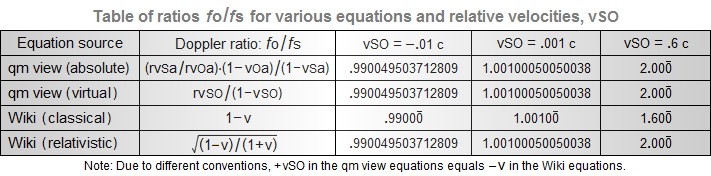The table shows that the orthodox, relativistic equation specifies the same Doppler shifts that the qm view shows are caused by the motions of light sources and observers through the qm.  The relativistic equation and the qm view, vSO equation are both good correlations between observed, virtual relative velocity and observed virtual Doppler shifts.  But they do not reveal what is causing the Doppler shifts.  Because the orthodox equations express Doppler shifts in terms of relative motion between source and observer, they contribute to the illusion that relative motion is causing Doppler shifts.  Orthodox theory cannot explain why relative motion between a source and an observer causes Doppler shifts.  On the other hand, the qm view identifies the physical causes for the Doppler shifts specified by its equations.  It shows that the causes of Doppler shifts of light, like the causes of "relativistic" phenomena in general, are more complex than relative motion between sources and observers.

Unless it can be shown that the above discussion is incorrect, this information is strong evidence supporting the view that light is propagated at a constant, in-vacuo speed through a quantum medium, and not at a constant speed relative to all sources and observers of light.  And given the fact that so much of modern physics theory depends on the soundness of the light speed, c, assumption, is it not important to be aware of the many reasons why light speed c is an illusion?  The incompatibility of light speed, c, and Doppler shifts of light frequencies is one of the reasons.